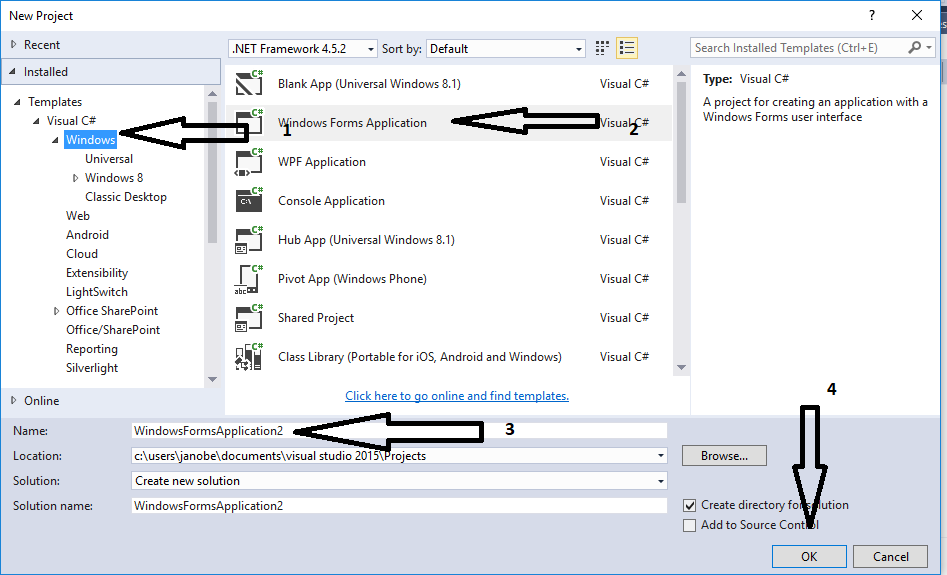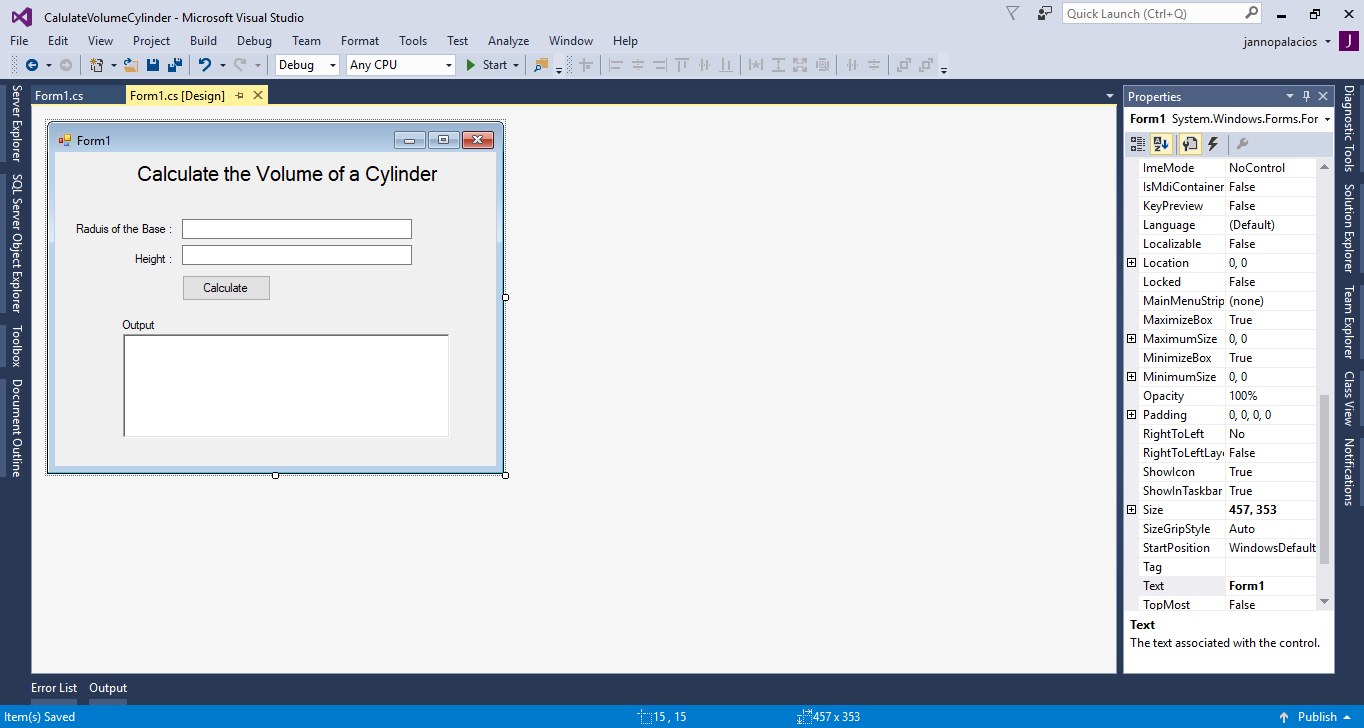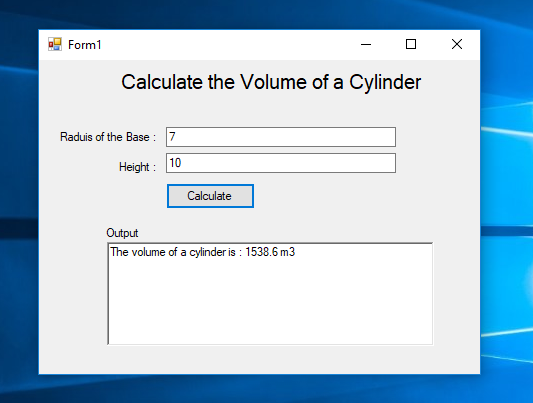# How to Get the Volume of a Cylinder Using C#

In this tutorial, I will teach you how to get the volume of a cylinder using c#. We all know that the formula for the volume of a cylinder is pi times radius squared times height. So. I made a program that can help you calculate the volume of a cylinder with ease. In order to use this program, you have to put the radius of the base and the height, then click the calculate button to get the result.

## Step 1

Open Microsoft Visual Studio 2015 and create a new windows form application for c#.## Step 2

Do the form just like shown below.## Step 3

Double click the calculate button to open the `button1_Click `event handler and do the following code for calculating the volume of a cylinder.
`         private void button1_Click(object sender, EventArgs e)        {            //declaring variables            double inp_based = 0;            double inp_height = 0;            double pi = 3.14;            double volume = 0;              //passing the value of a textbox in the variables            inp_based = double.Parse(txtBase.Text);            inp_height = double.Parse(txtHieght.Text);             // Set the formula to get the volume of a cylinder            volume = ((inp_based * inp_based) * inp_height) * pi;             //displaying the result            txtOutput.Text = "The volume of a cylinder is : " +  volume.ToString() + " m3";        }`
OutputThe complete sourcecode is included. You can download it and run it on your computer. For any questions about this article. You can contact me @ Email – [email protected] Mobile No. – 09305235027 – TNT Or feel free to comment below.